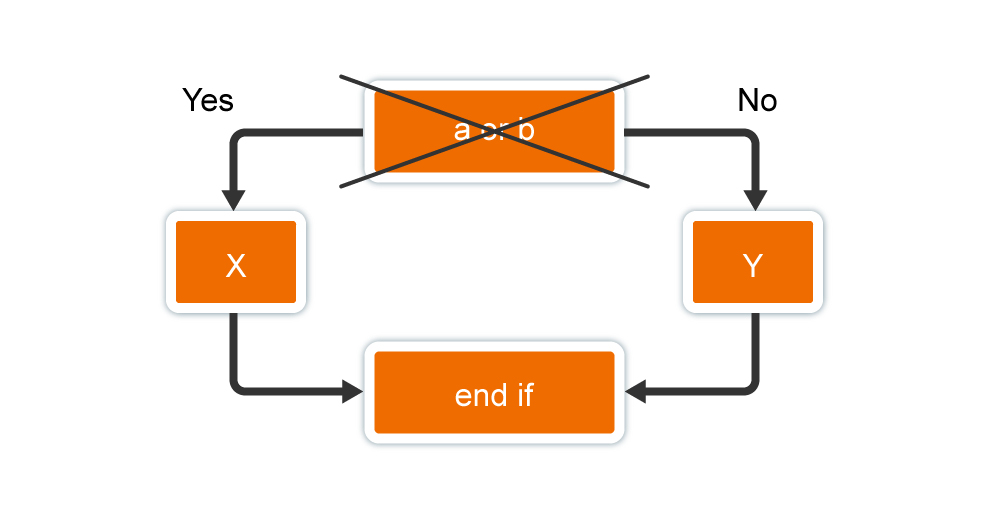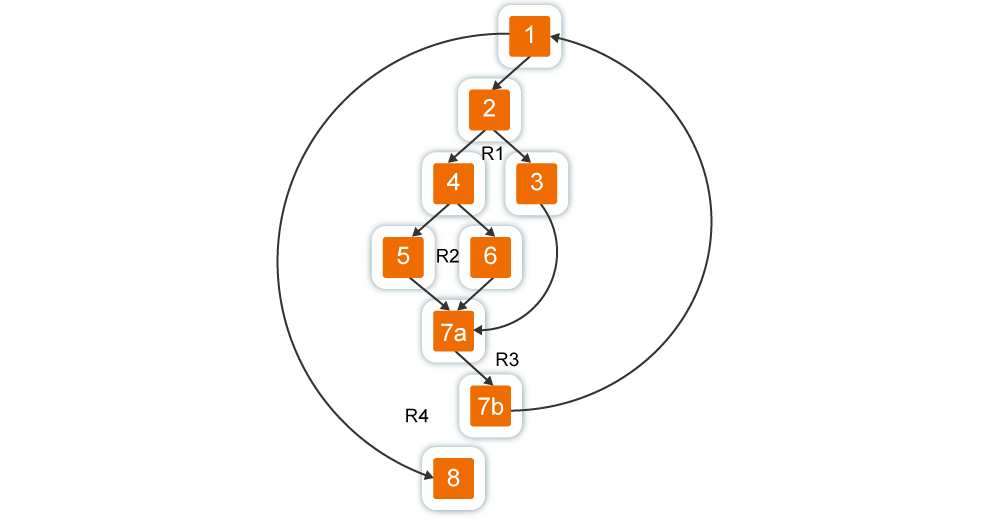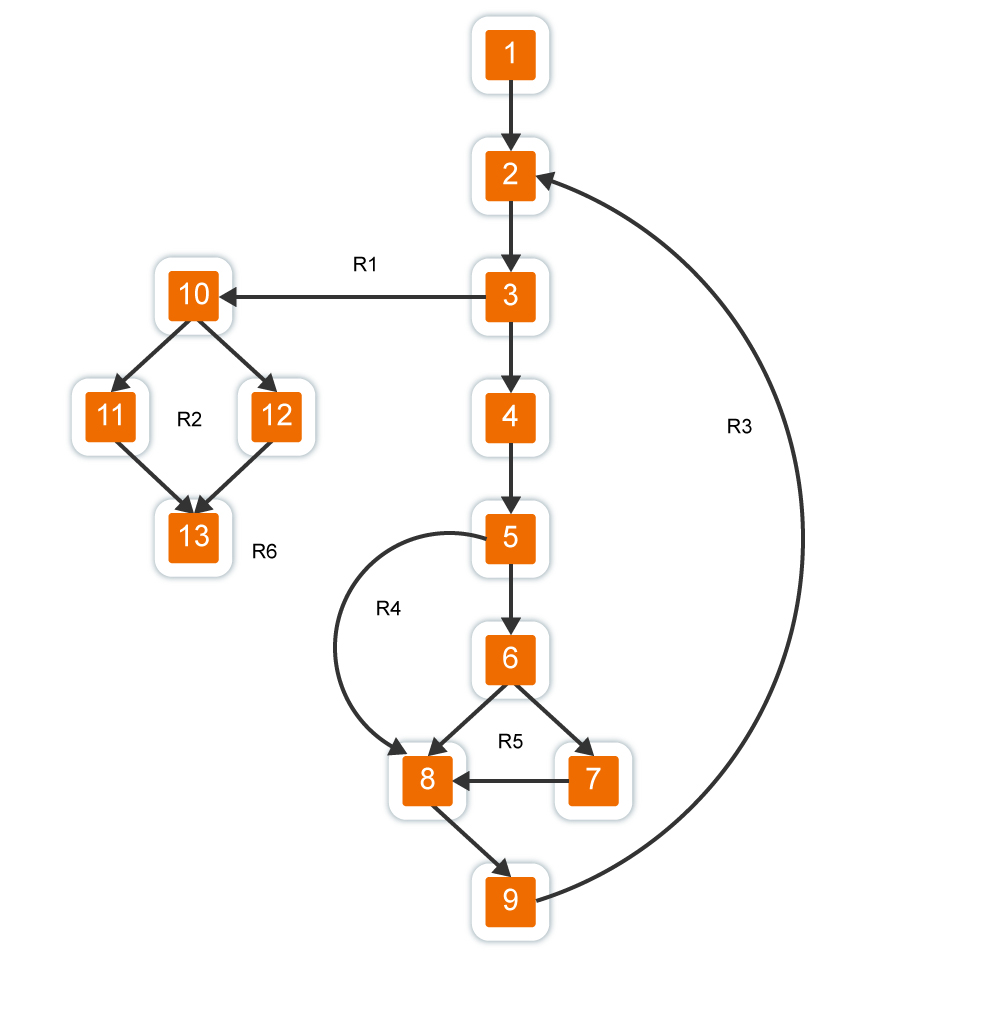# Basis path testingBasis path testing was mentioned in the article “Structural Testing”.  In this article we suggest to study the Basis path testing in more detail and consider its steps.

Basis path testing is a method based on the “white box” principle. The author of this method is Tom McCabe.

The Basis path testing allows you to:

– receive an estimation of system complexity of the software;

– use this estimation to determine the required number of test cases.

Test cases are developed to test the basic set of paths (routes) in the program.  They guarantee a single execution of each program operator during the testing.

### Stream Graph

A stream graph is used to represent the program. Let’s list its features.

1. The graph is constructed as a reflection of the program’s control structure. During the reflection, the closing brackets of conditional operators and loop operators (end if; end loop) are considered as separate (fictitious) operators.

2. The nodes (vertices) of the flow graph correspond to the linear sections of the program and include one or several program operators.

3. The flow graph arcs represent the control flow in the program (control transfers between operators). The arc is an oriented edge.

4. The nodes are divided into operator and predicate. One arc emerges from the operator node, and two arcs from the predicate one.

5. Predicate nodes correspond to simple conditions in the program. The compound condition of the program is displayed in several predicate nodes. A compound condition is a condition in which one or more Boolean operations (OR, AND) are used.

For example, the program fragment

if a OR b

then x

else у

end if;

instead of a direct display of the form shown in Fig. 1 in the stream graph is mapped to the transformed stream graph (Figure 2).Fig. 1. Direct mapping to a stream graphFig. 2. The transformed stream graph

6. Closed areas formed by arcs and nodes are called regions.

7. The environment surrounding the graph is considered as an additional region. For example, the graph above has three regions – R1, R2, R3.

Example 1. Consider the compression procedure:

compression procedure

1 perform until EOF;

2 if the entry is empty

3 then delete the entry:

4 otherwise, if the field a> = field b

5 then delete b;

6 otherwise, delete a;

7 а end if;

7 а end if;

7 b end perform;

8 end compression;Fig. 3. The transformed stream graph of the compression procedure

It is mapped to the stream graph shown in Fig. 3. We see that this steam graph has four regions.

### Steps For the Basis Path Testing

To illustrate the steps of this method, we use a specific program – the procedure for calculating the mean value:

procedure avg;

1 i := 1;

1 input := 0;

1 number := 0;

1 sum := 0;

exec while 2 -val( i ) <> stop and input <=500 – 3

4 input := input + 1;

if 5 -val( i ) >= min and val ( i ) <= max – 6

7 then number := number + 1;

7 sum := sum + val( i );

8 end if;

8 i := i + 1;

9 end exec;

10 if number > 0

11 then avg := sum / number;

12 else avg := stop;

13 end if;

13 end avg;

Note that the procedure contains compound conditions (in the header of the loop and the conditional statement). Elements of compound conditions are placed in a framework for clarity.

Step 1. The stream graph based on the program text is formed:

– text operators are numbered (operator numbers are shown in the text of the procedure);

– the numbered program text is displayed in the nodes and vertices of the stream graph (Fig. 4).Fig. 4. The stream graph of the average value calculating procedure

Step 2. The cyclomatic complexity of the stream graph is determined for each of the three formulas:

1) V (G) = 6 regions;

2) V (G) = 17 arcs – 13 nodes + 2 = 6;

3) V (G) = 5 predicate nodes + 1 = 6.

Step 3. The basic set of independent linear paths is defined:

Path 1: 1-2-10-11-13; /val=stор, number>0.

Path 2: 1-2-10-12-13;/val=stop, number=0.

Path 3: 1-2-3-10-11-13; /attempt to process the 501-th value.

Path 4: 1-2-3-4-5-8-9-2-… /val<min.

Path 5: 1-2-3-4-5-6-8-9-2-… /val>max.

Path 6: 1-2-3-4-5-6-7-8-9-2-… /normal processing mode.

NOTE

For the ease of further analysis, the launch conditions are indicated for each path. The full stops at the end of paths 4, 5, 6 indicate that any continuation through the remainder of the control structure of the graph is allowed.

Step 4. Test cases that initiate execution of each path are prepared.

Each test case is formed as follows:

Input data (ID)

Expected results (ER)

The input data must be selected in such a way that the predicate vertices provide the necessary switching: starting only those operators that are listed in a particular path in the required order.

Let’s define the test cases that answer the identified set of independent paths.

Test case for path 1 TC1:

ID: val(k) = permissible value, where k < i;val(i) = stop, where 2 < i < 500.

ER: correct averaging is based on k values and correct counting.

NOTE

The path can not be tested independently, but should be tested as part of paths 4, 5, 6 (difficulties in verifying the 11th operator).

Test case for path 2 TC2:

ID: val(1)=stор.

ER: avg=stор, other quantities have initial values.

Test case for path 3 TС3:

ID: attempt to process the 501-th value, the first 500 values must be correct.

ER: correct averaging is based on k values and correct counting.

Test case for path 4 TC4:

ID: val(i)=permissible value, where i ≤ 500; val(k) < min, where k < i.

ER: correct averaging is based on k values and correct counting.

Test case for path 5 TC5:

ID: val(i)=permissible value, where i ≤ 500; val(k) > max, where k < i.

ER: correct averaging is based on n quantities and correct counting

Test case for path 6 TC6:

ID: val(i)=permissible value, where i ≤ 500.

ER: correct averaging is based on n quantities and correct counting

The actual results of each test case are compared with the expected results. After all test cases are executed, it is guaranteed that all program operators are executed at least once.

It is important to note that some independent paths can not be inspected in isolation. Such paths must be tested when testing another path (as a part of another test case).